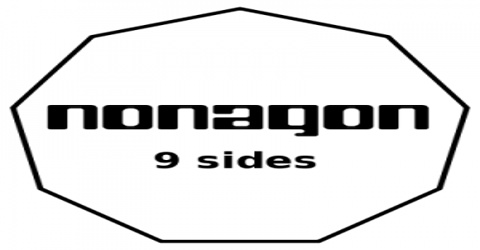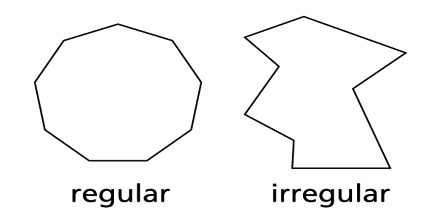Mathematic

# Nonagon PolygonNonagon is one of the types of polygonal shapes. The nonagon is said to be a 9 sided polygon. It is also called Enneagon. The polygon sides are always straight and it is said to be a two dimensional shape.Properties of regular nonagon:

• The interior angle of the regular polygon is 140 degree. If we find the interior angle by using the given formula, the formula is (180n – 360n)/n. (Here the value of n = 9).
• Exterior Angle is 40°. To find the exterior angle of a regular decagon, we use the fact that the exterior angle forms a linear pair with the interior angle, so in general it is given by the formula 180-interior angle.
• The number of diagonals in nonagon is 27, and number of triangles in the nonagon is 7.
• Perimeter of the nonagon = 9a, where “a” is side length of nonagon.

Regular Nonagons

A regular polygon is a polygon with equal sides and equal angles. Regular nonagon is a nine-sided shape with equal sides and equal angles of 140 degrees each.Irregular Nonagons

An irregular polygon is a polygon with unequal sides and angles. An irregular nonagon is a nine-sided shape that does not have equal sides or angles.